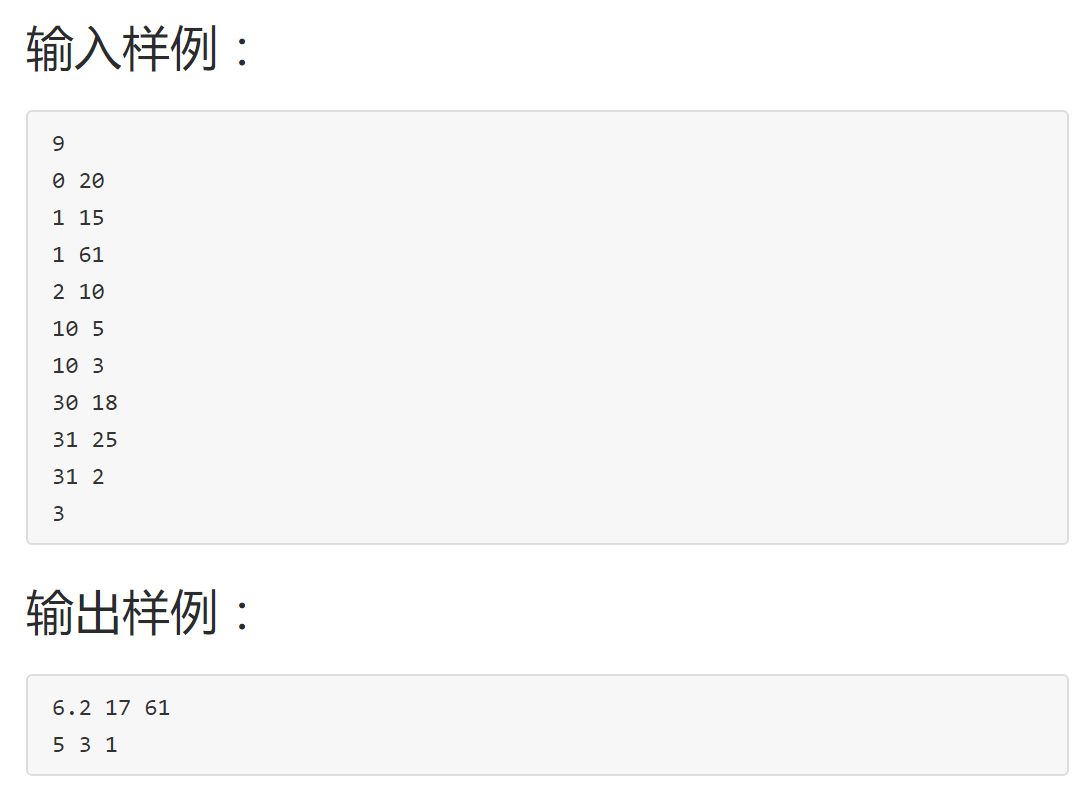习题8.1 银行排队问题之单队列多窗口服务

输出格式:#include<stdio.h>
#include<stdlib.h>
typedef struct node
{
int t, p;//到达时间，处理时间
}ST;
ST q;//数组模拟队列
int main()
{
int l, r, n, k, i;
while(~scanf("%d", &n))
{
l = r = 0;//队列头和尾
for(i = 0; i < n; i++)
{
scanf("%d %d", &q[r].t, &q[r].p);//将输入的数入队列
if(q[r].p > 60) q[r].p = 60;//根据题目要求，最大处理时间60
r++;
}
scanf("%d", &k);//k个窗口
int sumwait = 0, lenwait = 0, wait = 0;//总的等待时间， 最长的等待时间， 单次等待时间
int sum = {0}, winnum = {0};//完成时间，窗口人数
while(l < r)
{
int flag = 0, minn = 99999, imin = 0;//标记变量， 最快的完成时间， 最快完成时间的下标
for(i = 0; i < k; i++)//遍历k个窗口
{
if(sum[i] < q[l].t)//如果队列首位，到达时间比，完成时间大，就代表不需要等待
{
sum[i] = q[l].t + q[l].p;//更新完成这个窗口完成的时间
winnum[i]++;//窗口人数加一
flag = 1;//标记一下，代表不需要等待
l++;//队列首位除去
break;//退出循环
}
if(minn > sum[i])//如果需要等待，就记录各个窗口里最快完成的那个窗口的完成时间，和下标
{
minn = sum[i];
imin = i;
}
}
if(!flag)//需要等待
{
wait = minn - q[l].t;//等待的时间，最快完成的时间减去队列第一个人到达的时间
if(lenwait < wait) lenwait = wait;//不断更新等待的最长时间
sumwait += wait;//求等待时间的和
sum[imin] = minn + q[l].p;//更新对应窗口的完成时间
winnum[imin]++;//对应窗口人数++
l++;//队列删除首位
}
}
int last = 0;
for(i = 0; i < k; i++)
{
if(last < sum[i]) last = sum[i];//求最大完成时间
}
printf("%.1lf %d %d\n", 1.0 * sumwait / n, lenwait, last);//输出，平均等待时间， 最长等待时间， 最后完成时间
for(i = 0; i < k; i++)
{
printf("%d", winnum[i]);//输出各个窗口的人数
if(i == k - 1) printf("\n");
else printf(" ");
}
}
return 0;
}